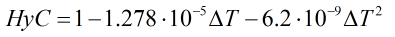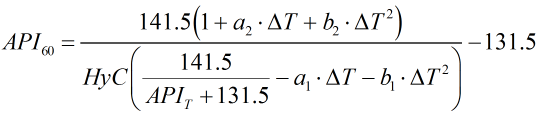# C197 – ASTM-IP Table 5:1952

## Description

This calculates the API gravity at 60°F from an observed API gravity and corresponding temperature.

Report on the Development, Construction, Calculation and Preparation of the ASTM-IP Petroleum Measurement Tables – ASTM D 1250; IP 200 (1960)

ASTM-IP Petroleum Measurement Tables – ASTM D 1250; IP 200 (1952)

Kelton calculation reference C197

FLOCALC calculation reference F083

## Options

### Precision

• Full
• Rounding

This option is used to choose whether to apply rounding to the same number of significant figures as the printed values in Petroleum Measurement Table 5 or output values with full precision.

### Hydrometer correction

• Apply

This option is chosen to apply the hydrometer correction described below.

## Calculation

### Hydrometer Correction

The hydrometer correction is calculated by:Where ΔT = Difference in temperature compared to base temperature in °F

### API Gravity at 60°F

The API gravity at 60°F is calculated by an iterative process based around the relationship:Where ΔT = Difference in temperature compared to base temperature in °F APIT = API gravity at observed temperature a1 = Constant from Table III a2 = Constant from Table III b1 = Constant from Table III b2 = Constant from Table III

Back